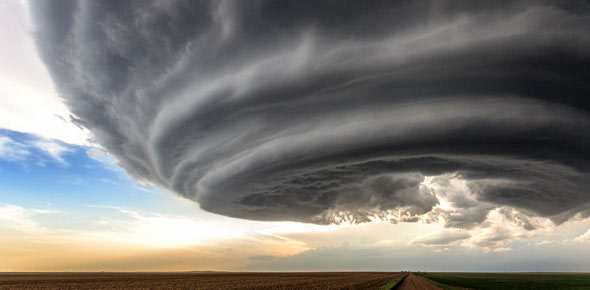# Physical Science Benchmark #1 Review

77 Questions | Attempts: 148Settings• 1.
If an object has kinetic energy, then it also must have _____.
• A.

Speed

• B.

Impulse

• C.

Acceleration

• D.

Force

• 2.
An object that has kinetic energy must be _____.
• A.

Moving.

• B.

Falling.

• C.

At an elevated position.

• D.

At rest.

• 3.
The formula to calculate work is   W= F x d How much work was done is 5 N of force was used to move an object 25 meters?
• A.

30 Joules

• B.

125 Joules

• C.

5 Joules

• D.

20 Joules

• 4.
The formula for calculating work = W= F x d A vehicle at the wrecking yard was pushed forward 7 meters with a force of 5000 N.  How much work was done?
• A.

5007 J

• B.

4993 J

• C.

35000 J

• D.

714.2 J

• 5.
The formula for calculating work is F x d A family car ran out of gas in the left lane of a busy street.  The father got out of the car and tried to push the car to the gas station.  He used about 600 N of force, but the car moved a distance of 0.  How much work was done?
• A.

600 J

• B.

1200 J

• C.

300 J

• D.

0 J

• 6.
According to the definition for work, in order for work to be accomplished their must be a force exerted on an object and
• A.

The object must move in the opposite direction of the applied force

• B.

The object must move in the same direction as the applied force

• C.

The object must be lifted up

• D.

The object must move a distance less than 100 meters

• 7.
The formula for calculating work is W= F x d A pulley is used to lift a heavy piano 6 meters up to the second floor.  The amount of force applied upward is 700 N.  How much work was done?
• A.

706 J

• B.

4200 J

• C.

116.6 J

• D.

694 J

• 8.
The formula for calculating work is W= F x d Jerry pulled the library cart 3 meters with a force of 17 N.  How much work was done?
• A.

51 J

• B.

20 J

• C.

14 J

• D.

5.6 J

• 9.
The formula for calculating work is W= F x d. A body builder lifts the barbell up 1 meter with a force of 501 N. Then He uses 501 N to hold the barbell in place for 2 minutes. How much work was done?
• A.

1002 J

• B.

501 J

• C.

500 J

• D.

250. 5 J

• 10.
The ability to do work or cause change is...
• A.

Work

• B.

Gravity

• C.

Energy

• 11.
The energy an object has while in motion is...
• A.

Elastic potential energy

• B.

Gravitational potential energy

• C.

Kinetic energy

• 12.
Stored energy that results from the position or shape of an object is called...
• A.

Potential energy

• B.

Kinetic energy

• C.

Thermal energy

• 13.
Calculate the momentum of a 700g ball that is rolling down a ramp at 4.6m/s.
• A.

3.22 kg x m/s

• B.

3,220 kg x m/s

• C.

152.17 kg x m/s

• D.

6.57 kg x m/s

• 14.
If a force of 50N acts on a 5 kg object, its acceleration is equal to ______ meters per second squared.
• A.

55

• B.

45

• C.

250

• D.

10

• 15.
The resistance an object has to a change in its motion is called ______________,
• A.

Equilbrium

• B.

Inertia

• C.

Momentum

• D.

Drag

• 16.
3. If a total distance of 750 m is covered in a time interval of 25 s,the average speed is ______?
• A.

3,974 mph

• B.

3 mph

• C.

30 mph

• D.

30 m/s

• 17.
6. If a person walked at 2 m/s for 12 s he/she would travel a distance of ________.
• A.

24 m

• B.

6 m

• C.

4 m

• D.

• 18.
7. How long would it take to travel 50 km traveling at a speed of 10 km/hr?
• A.

3 hours

• B.

1 hour

• C.

5 hours

• D.

50 hours

• 19.
The current in a circuit equals the voltage difference divided by the resistance.
• A.

Law of resistance

• B.

Law of compliance

• C.

Ohms Law

• D.

Conductivity Law

• E.

Static electricity

• 20.
The net movement of electric charges in a singe direction.
• A.

Voltage difference

• B.

Electric current

• C.

Electric circuits

• D.

Batteries

• 21.
A  ____ ____ is related to the force that causes electric charges to flow.
• A.

Electric currents

• B.

Voltage difference

• C.

Dry cell batteries

• D.

Current flow

• 22.
Voltage difference is measured in _____.
• A.

Amps

• B.

Electrodes

• C.

Volts

• D.

Centimeters

• 23.
What is the unit for voltage?
• A.

Volts

• B.

Amperes

• C.

Ohms

• D.

Joules

• 24.
What is the unit for current?
• A.

Volts

• B.

Amperes

• C.

Ohms

• D.

Joules

• 25.
What is the unit for resistance?
• A.

Volts

• B.

Amperes

• C.

Ohms

• D.

Joules

## Related TopicsBack to top
×

Wait!
Here's an interesting quiz for you.